﻿ 分子机器中链状分子热膨胀性质的理论研究 Theoretical Study on Thermal Expansions of Chain Molecules in Molecular Machines

Modern Physics
Vol.07 No.06(2017), Article ID:22597,6 pages
10.12677/MP.2017.76025

Theoretical Study on Thermal Expansions of Chain Molecules in Molecular Machines

Jing Tang1, Jianping Huang2*

1College of Physics and Information Science, Hunan Normal University, Changsha Hunan

2College of Information Science and Technology, Hunan Normal University, Changsha Hunan

*通讯作者。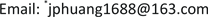Received: Oct. 20th, 2017; accepted: Nov. 1st, 2017; published: Nov. 7th, 2017ABSTRACT

The formulas to describe thermal expansions of interatomic distances and thermal expansion coefficients of chain molecules in molecular machines were derived based on the lattice dynamics, and then the numerical calculations were carried out. The numerical results show that at the low temperature, when the length of short chain molecule increases, the thermal expansion of interatomic distance increases rapidly and the thermal expansion coefficient decreases rapidly, and they approach to the corresponding values of the chain molecule with infinite length rapidly. Therefore, there are size effects in the thermal expansion properties of chain molecules; the size effects of thermal expansion properties of chain molecules are weakened obviously when temperature increase and finally disappeared at high temperature limit. It is concluded that the size effects of thermal expansion properties of chain molecules must be considered at low temperature.

Keywords:Chain Molecule, Thermal Expansion, Lattice Dynamics, Perturbation Theory, Size Effect

1湖南师范大学物理与信息科学学院，湖南 长沙

2湖南师范大学信息科学与工程学院，湖南 长沙1. 引言

2. 链状分子的晶格动力学

${u}_{l}=\underset{k}{\sum }{A}_{k}{\text{e}}^{i\left({\omega }_{k}t-kal\right)}$ (1)

${\omega }_{k}^{2}=\frac{4\beta }{m}{\mathrm{sin}}^{2}\frac{ka}{2}$ (2)

${u}_{l}=\underset{k}{\sum }{A}_{k}\mathrm{cos}\left(kal+{\varphi }_{k}\right){\text{e}}^{i{\omega }_{k}t}$ (3)

${u}_{l}=\underset{j=1}{\overset{N-1}{\sum }}{A}_{j}\mathrm{cos}\left(l-\frac{1}{2}\right)\frac{j\text{π}}{N}{\text{e}}^{i{\omega }_{j}t}$ (4)

${\omega }_{j}^{2}=4\frac{\beta }{m}{\mathrm{sin}}^{2}\frac{j\text{π}}{2N}$ (5)

${u}_{l}=\underset{j=1}{\overset{N-1}{\sum }}\sqrt{\frac{\hslash }{N{m}_{a}{\omega }_{j}}}\left({a}_{j}+{a}_{j}^{+}\right)\mathrm{cos}\left(l-\frac{1}{2}\right)\frac{j\text{π}}{N}$ (6)

${p}_{l}=-i\underset{j=1}{\overset{N-1}{\sum }}\sqrt{\frac{\hslash m{\omega }_{j}}{N}}\left({a}_{j}-{a}_{j}^{+}\right)\mathrm{cos}\left(l-\frac{1}{2}\right)\frac{j\text{π}}{N}$ (7)

${H}_{0}=\underset{k}{\sum }\left({n}_{k}+\frac{1}{2}\right)\hslash {\omega }_{k}$ (8)

${\stackrel{¯}{n}}_{k}=\frac{1}{{\text{e}}^{\hslash {\omega }_{k}/{k}_{B}T}-1}$ (9)

3. 链状分子的热膨胀

${H}^{\prime }=\underset{p\ge 3}{\sum }\underset{l=1}{\overset{N-1}{\sum }}\frac{{f}_{p}}{p!}{\left({u}_{l+1}-{u}_{l}\right)}^{p}$ (10)

${\Delta }_{p}^{chain}a\left(l+1,l\right)=2\mathrm{Re}\underset{{n}^{\prime }\ne n}{\sum }\frac{〈{n}^{\prime }|{H}_{p}|n〉}{{E}_{n}-{E}_{n\text{'}}}〈n|{u}_{l+1}-{u}_{l}|{n}^{\prime }〉$ (11)

$|n〉=|{n}_{{k}_{1}},\cdots ,{n}_{{k}_{j}}\cdots 〉$ (12)

$|{n}^{\prime }〉=|{n}_{{k}_{1}},\cdots ,{n}_{{k}_{j}}±1\cdots 〉$ (13)

${\Delta }_{p}^{chain}a\left(l+1,l\right)=-\frac{{f}_{p}}{\beta \left(p-1\right)!}〈{n}_{{k}_{1}},{n}_{{k}_{2}},\cdots {n}_{{k}_{N-1}}|{\left({u}_{l+1}-{u}_{l}\right)}^{p-1}|{n}_{{k}_{1}},{n}_{{k}_{2}},\cdots {n}_{{k}_{N-1}}〉$ (14)

$p=2\lambda +1$ ，且 $\lambda =1,2,\cdots$ ，即p为奇数时，由(14)式得

${\Delta }_{2\lambda +1}^{chain}a\left(l+1,l\right)=-\frac{{f}_{2\lambda +1}}{{2}^{\lambda }\lambda !\beta }{〈{n}_{{k}_{1}},{n}_{{k}_{2}},\cdots ,{n}_{{k}_{N-1}}|{\left({u}_{l+1}-{u}_{l}\right)}^{2}|{n}_{{k}_{1}},{n}_{{k}_{2}},\cdots {n}_{{k}_{N-1}}〉}^{\lambda }$ (15)

${\Delta }_{2\lambda +1}^{chain}a\left(l+1,l\right)=-\frac{{f}_{2\lambda +1}}{{2}^{\lambda }\lambda !{\beta }^{\lambda +1}}{\left[\frac{2}{N}\underset{j=1}{\overset{N-1}{\sum }}\left({\stackrel{¯}{n}}_{j}+\frac{1}{2}\right)\hslash {\omega }_{j}{\mathrm{sin}}^{2}\frac{jl\text{π}}{N}\right]}^{\lambda }$ (16)

4. 数值计算结果与分析

5. 结论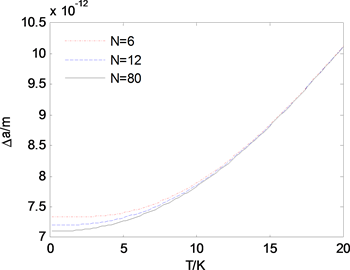Figure 1. Thermal expansion of interatomic spacing in chain molecule vs. temperature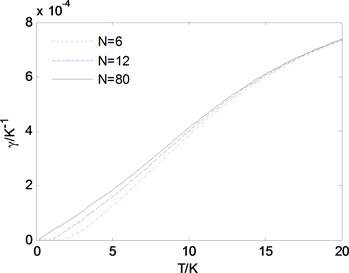Figure 2. Thermal expansion coefficient of chain molecule vs. temperature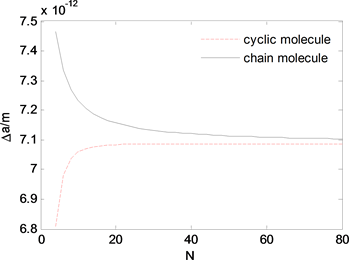Figure 3. Thermal expansion of interatomic spacing in chain molecule vs. its length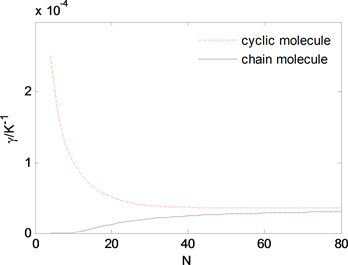Figure 4. Thermal expansion coefficient of chain molecule vs. its length

Theoretical Study on Thermal Expansions of Chain Molecules in Molecular Machines[J]. 现代物理, 2017, 07(06): 221-226. http://dx.doi.org/10.12677/MP.2017.76025

1. 1. Cheng, C. and Stoddart, J.F. (2016) Wholly Synthetic Molecular Machine. Materials Chemistry and Physics, 17, 1780- 1793. https://doi.org/10.1002/cphc.201501155

2. 2. Anelli, P.L., Spencer, N. and Stoddart, J.F. (1991) A Molecular Shuttle. Journal of the American Chemical Society, 113, 5131-5133. https://doi.org/10.1021/ja00013a096

3. 3. Badjic, J.D., Ronconi, C.M., Stoddart, J.F., et al. (2015) Operating Molecular Elevators. Journal of the American Chemical Society, 128, 1489-1499. https://doi.org/10.1021/ja0543954

4. 4. Liu, Y., Flood, A. H., Bonvallet, P.A., et al. (2005) Linear Artificial Molecular Muscles. Journal of the American Chemical Society, 127, 9745-9759. https://doi.org/10.1021/ja051088p

5. 5. Koumura, N., Zijlstra, R.W., Van Delden, R.A., et al. (1999) Light-Driven Monodirectional Molecular Rotor. Nature, 401, 152-155. https://doi.org/10.1038/43646

6. 6. Ruangsupapichat, N., Pollard, M.M., Harutyunyan, S.R., et al. (2011) Reversing the Direction in a Light-Driven Rotary Molecular Motor. Nature Chemistry, 3, 53-60. https://doi.org/10.1038/nchem.872

7. 7. Kudernac, T., Ruangsupapichat, N., Parschau, M., et al. (2011) Electrically Driven Directional Motion of a Four-Wheeled Molecule on a Metal Surface. Nature, 479, 208-211. https://doi.org/10.1038/nature10587

8. 8. 唐婧, 黄建平. 分子机器中环状分子热膨胀性质的理论研究[J]. 现代物理, 2017, 7(5): 163-168.

9. 9. Bottger, H. (1983) Principles of the Theory of Lattice Dynamics. Weinheim: Physik Verlag, 85-90.

10. 10. Huang, J.P., Wu, X.Z. and Li, S.Y. (2005) Thermal Expansion Coefficients of Thin Crystal Films. Communications in Theoretical Physics, 44, 921-924. https://doi.org/10.1088/6102/44/5/921